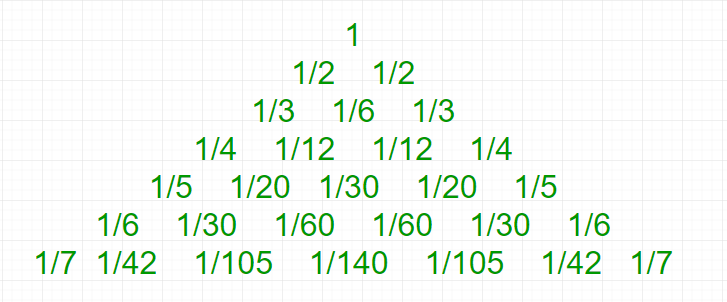Related Articles

# Leibniz harmonic triangle

• Difficulty Level : Medium
• Last Updated : 13 Apr, 2021

The Leibniz harmonic triangle is a triangular arrangement of unit fractions in which the outermost diagonals consist of the reciprocals of the row numbers and each inner cell is the cell diagonally above and to the left minus the cell to the left. To put it algebraically, L(r, 1) = 1/r, where r is the number of the row, starting from 1, and c is the number, never more than r and L(r, c) = L(r – 1, c – 1) – L(r, c – 1)Relation with pascal’s triangle
Whereas each entry in Pascal’s triangle is the sum of the two entries in the above row, each entry in the Leibniz triangle is the sum of the two entries in the row below it. For example, in the 5th row, the entry (1/30) is the sum of the two (1/60)s in the 6th row.
Just as Pascal’s triangle can be computed by using binomial coefficients, so can Leibniz’s:Properties
If one takes the denominators of the nth row and adds them, then the result will equal n.2n-1. For example, for the 3rd row, we have 3 + 6 + 3 = 12 = 3 × 22.
Given a positive integer n. The task is to print Leibniz harmonic triangle of height n.
Examples:

Input : n = 4
Output :
1
1/2 1/2
1/3 1/6 1/3
1/4 1/12 1/12 1/4

Input : n = 3
Output :
1
1/2 1/2
1/3 1/6 1/3

Below is the implementation of printing Leibniz harmonic triangle of height n based on above relation with Pascal triangle.

## C++

 // CPP Program to print Leibniz Harmonic Triangle#include using namespace std; // Print Leibniz Harmonic Trianglevoid LeibnizHarmonicTriangle(int n){    int C[n + 1][n + 1];     // Calculate value of Binomial Coefficient in    // bottom up manner    for (int i = 0; i <= n; i++) {        for (int j = 0; j <= min(i, n); j++) {             // Base Cases            if (j == 0 || j == i)                C[i][j] = 1;             // Calculate value using previously            // stored values            else                C[i][j] = C[i - 1][j - 1] + C[i - 1][j];        }    }     // printing Leibniz Harmonic Triangle    for (int i = 1; i <= n; i++) {        for (int j = 1; j <= i; j++)            cout << "1/" << i * C[i - 1][j - 1] << " ";         cout << endl;    }} // Driven Programint main(){    int n = 4;    LeibnizHarmonicTriangle(n);    return 0;}

## Java

 // Java Program to print// Leibniz Harmonic Triangleimport java.io.*;import java.math.*; class GFG {         // Print Leibniz Harmonic Triangle    static void LeibnizHarmonicTriangle(int n)    {        int C[][] = new int[n + 1][n + 1];             // Calculate value of Binomial        // Coefficient in bottom up manner        for (int i = 0; i <= n; i++) {            for (int j = 0; j <= Math.min(i, n);                                          j++) {                     // Base Cases                if (j == 0 || j == i)                    C[i][j] = 1;                     // Calculate value using                // previously stored values                else                    C[i][j] = C[i - 1][j - 1] +                              C[i - 1][j];            }        }             // printing Leibniz Harmonic Triangle        for (int i = 1; i <= n; i++) {            for (int j = 1; j <= i; j++)                System.out.print("1/" + i * C[i - 1][j - 1]                                          + " ");                 System.out.println();        }    }         // Driven Program    public static void main(String args[])    {        int n = 4;        LeibnizHarmonicTriangle(n);    }} // This code is contributed by Nikita Tiwari

## Python3

 # Python3 Program to print# Leibniz Harmonic Triangle # Print Leibniz Harmonic# Triangledef LeibnizHarmonicTriangle(n):    C = [[0 for x in range(n + 1)]            for y in range(n + 1)];                 # Calculate value of Binomial    # Coefficient in bottom up manner    for i in range(0, n + 1):        for j in range(0, min(i, n) + 1):                         # Base Cases            if (j == 0 or j == i):                C[i][j] = 1;                             # Calculate value using            # previously stored values            else:                C[i][j] = (C[i - 1][j - 1] +                           C[i - 1][j]);                               # printing Leibniz    # Harmonic Triangle    for i in range(1, n + 1):        for j in range(1, i + 1):            print("1/", end = "");            print(i * C[i - 1][j - 1],                           end = " ");        print(); # Driver CodeLeibnizHarmonicTriangle(4); # This code is contributed# by mits.

## C#

 // C# Program to print Leibniz Harmonic Triangleusing System; class GFG {         // Print Leibniz Harmonic Triangle    static void LeibnizHarmonicTriangle(int n)    {        int [,]C = new int[n + 1,n + 1];             // Calculate value of Binomial        // Coefficient in bottom up manner        for (int i = 0; i <= n; i++) {            for (int j = 0; j <= Math.Min(i, n);                                        j++) {                     // Base Cases                if (j == 0 || j == i)                    C[i,j] = 1;                     // Calculate value using                // previously stored values                else                    C[i,j] = C[i - 1,j - 1] +                            C[i - 1,j];            }        }             // printing Leibniz Harmonic Triangle        for (int i = 1; i <= n; i++) {            for (int j = 1; j <= i; j++)                Console.Write("1/" + i * C[i - 1,j - 1]                                        + " ");             Console.WriteLine();        }    }         // Driven Program    public static void Main()    {        int n = 4;                 LeibnizHarmonicTriangle(n);    }} // This code is contributed by vt_m.

## PHP

 

## Javascript

 

Output:

1/1
1/2 1/2
1/3 1/6 1/3
1/4 1/12 1/12 1/4 

Attention reader! Don’t stop learning now. Get hold of all the important DSA concepts with the DSA Self Paced Course at a student-friendly price and become industry ready.  To complete your preparation from learning a language to DS Algo and many more,  please refer Complete Interview Preparation Course.

In case you wish to attend live classes with experts, please refer DSA Live Classes for Working Professionals and Competitive Programming Live for Students.

My Personal Notes arrow_drop_up